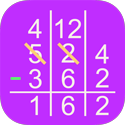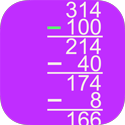# Math App Bundles

## Visual Fraction and Multiplication Table Bundle

Your savings: 53 %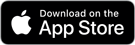Visual Fractions Decimals and Percentages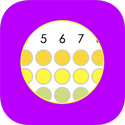Visual Multiplication TableFraction as Slope

## Math Division, Multiplication, Addition, Subtraction, Fractions, and Rounding

Your savings: 50 %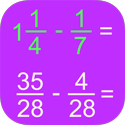Fraction MathLong Division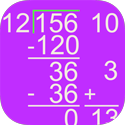Partial Quotients Division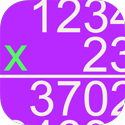Long MultiplicationPartial Products Multiplication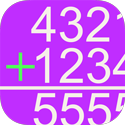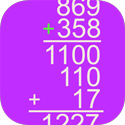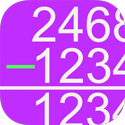Column SubtractionDecimal RoundingWhole Number Rounding

## Order of Operations and Polynomial Algebra Bundle

Your savings: 55 %Order of OperationsPolynomial Long Division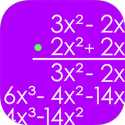Polynomial Multiplication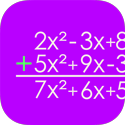Polynomial Addition and Subtraction

## Alternative Multiplication and Addition Algorithms Bundle

Your savings: 57 %Vertically and Crosswise Multiplication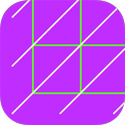Lattice MultiplicationGrid MultiplicationRussian Multiplication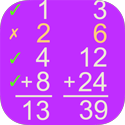Egyptian Multiplication

## Alternative Division and Subtraction Algorithms Bundle

Your savings: 57 %Same-Change SubtractionColumn Division

Counting-Up SubtractionPartial Differences Subtraction